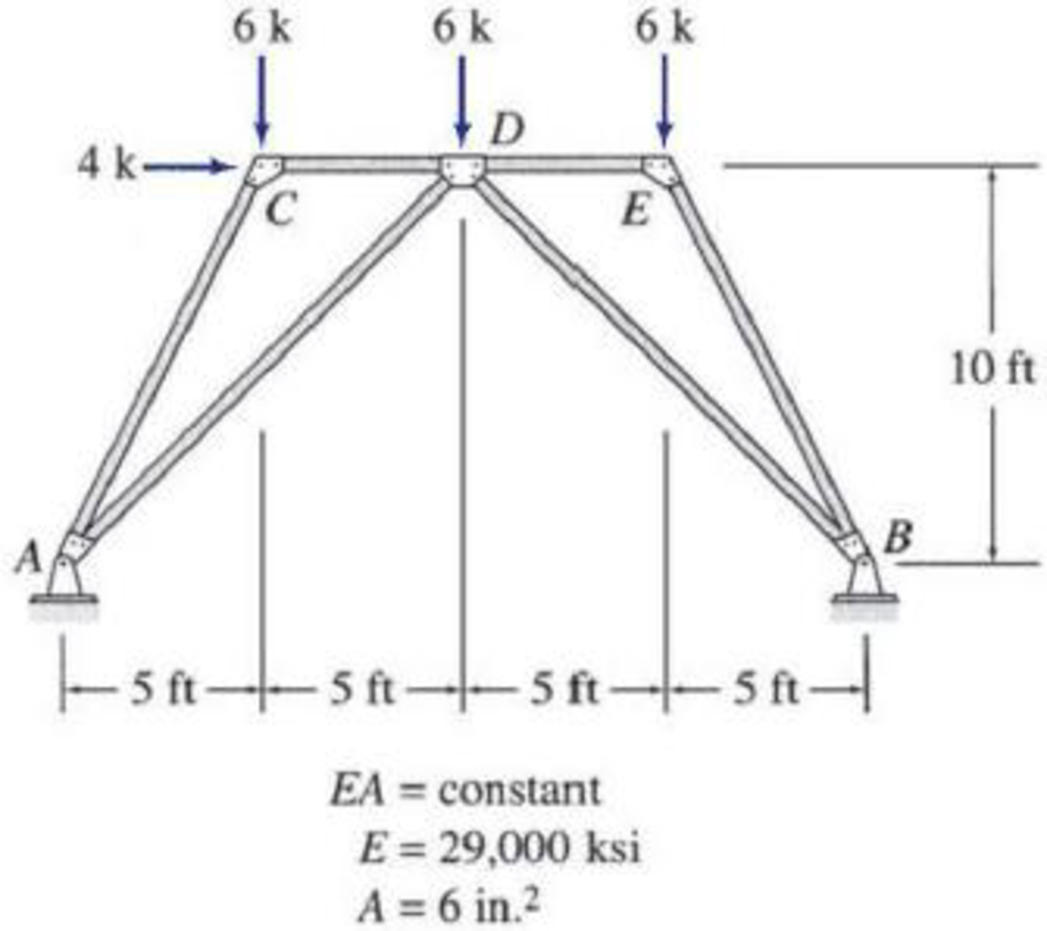# Use Castigliano’s second theorem to determine the horizontal deflection at joint E of the truss shown in Fig. P7.8. FIG. P7.8, P7.56

#### Solutions

Chapter
Section
Chapter 7, Problem 56P
Textbook Problem
103 views

## Use Castigliano’s second theorem to determine the horizontal deflection at joint E of the truss shown in Fig. P7.8.FIG. P7.8, P7.56To determine

Calculate the horizontal deflection at joint E of the truss.

### Explanation of Solution

Given information:

The truss is given in the Figure.

The value of E is 29,000 ksi and A is 6in.2

Apply the sign conventions for calculating reactions, forces and moments using the three equations of equilibrium as shown below.

• For summation of forces along x-direction is equal to zero (Fx=0), consider the forces acting towards right side as positive (+) and the forces acting towards left side as negative ().
• For summation of forces along y-direction is equal to zero (Fy=0), consider the upward force as positive (+) and the downward force as negative ().
• For summation of moment about a point is equal to zero (Matapoint=0), consider the clockwise moment as negative and the counter clockwise moment as positive.

Method of joints:

The negative value of force in any member indicates compression (C) and the positive value of force in any member indicates tension (T).

Condition for zero force members:

1. 1. If only two non-collinear members are connected to a joint that has no external loads or reactions applied to it, then the force in both the members is zero.
2. 2. If three members, two of which are collinear are connected to a joint that has no external loads or reactions applied to it, then the force in non-collinear member is zero.

Calculation:

Let apply a horizontal load P at joint B in the horizontal direction to find the horizontal deflection.

The value of load P is 0.

Let Ax and Ay be the horizontal and vertical reactions at the hinged support A.

Let Bx and By be the horizontal and vertical reactions at the hinged support B.

Sketch the resultant truss diagram for horizontal load P at joint E as shown in Figure 1.

Find the reactions at the supports using equilibrium equations:

Summation of moments about A is equal to 0.

MA=0By(20)6(15)6(10)6(5)4(10)P(10)=020By=220+10PBy=11+P2

Summation of forces along y-direction is equal to 0.

+Fy=0Ay+By666=0Ay+11+P2666=0Ay=7P2

Consider ACD, Summation of moments about D is equal to 0.

MD=0Ay(10)+Ax(10)+6(5)=0(7P2)(10)+Ax(10)+6(5)=0Ax=4P2

Summation of forces along x-direction is equal to 0.

+Fx=0AxBx+4=04P2Bx+4=0Bx=8+P2

Find the member forces using method of joints:

Apply equilibrium equation to the joint C:

Summation of forces along y-direction is equal to 0.

+Fy=06FACsin63.435°=0FAC=6.71k

Summation of forces along x-direction is equal to 0.

+Fx=04+FCDFACcos63.435°=04+FCD(6.71)cos63.435°=0FCD=7k

Apply equilibrium equation to the joint E:

Summation of forces along y-direction is equal to 0.

+Fy=06FBEsin63.435°=0FBE=6.71k

Summation of forces along x-direction is equal to 0.

+Fx=0PFDE+FBEcos63.435°=0PFDE+(6

### Still sussing out bartleby?

Check out a sample textbook solution.

See a sample solution

#### The Solution to Your Study Problems

Bartleby provides explanations to thousands of textbook problems written by our experts, many with advanced degrees!

Get Started

Find more solutions based on key concepts
Identify and list at least five different materials that are used in a TV set or computer.

Engineering Fundamentals: An Introduction to Engineering (MindTap Course List)

Describe the purpose of a pointing device. Give an example.

Enhanced Discovering Computers 2017 (Shelly Cashman Series) (MindTap Course List)

List three methods of applying cutting fluids.

Precision Machining Technology (MindTap Course List)

If your motherboard supports ECC DDR3 memory, can you substitute non-ECC DDR3 memory?

A+ Guide to Hardware (Standalone Book) (MindTap Course List)

Give one advantage of using electrodes with cellulose-based fluxes.

Welding: Principles and Applications (MindTap Course List)